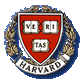# Math 1a - Introduction to Calculus Fall 2004 Resources

Home
Course Info
Sections
Resources
Schedule (MWF)
Schedule (TuTh)
HW Solutions
Exams
Worksheets
Precalc
Math Dept.

## Online Resources

• Definite Integrals: Here is a numerical integration applet which demonstrates how one can approximate a definite integral using left- and right-hand sums. The applet allows you to input a function, interval of integration (x0 to xn), and number of sub-intervals. You can also see three other approximation techniques. The midpoint technique we have seen; the trapezoid and Simpson's Rule techniques you will see in Math 1b.

Here is another, similar applet, and here is a Quicktime movie demonstrating the second applet.

• Newton's Method: You might find this Newton's Method applet useful. It demonstrates Newton's Method graphically for a certain function. You get to select the inital guess. The applet was written by Dan Sloughter of the Furman University Mathematics Department.

• Definition of the Derivative: Here is a tangent line applet that is designed to help you see how the two different versions of the definition of the derivative relate to tangent and secant line slopes.

• Transformations of Functions: The National Library of Virtual Manipulatives for Interactive Mathematics has a graphing tool that is useful for visualizing transformations of functions. One can graph a function like f(x) = a | x | + b, then use sliders to vary the parameters a and b and see what effect they have on the graph of the function.

• Visual Calculus:: Visual Calculus is a collection of calculus tutorials developed by the University of Tennessee, Knoxville, Mathematics Department. You may find some of the tutorials helpful as you study, particularly the ones featuring animations.

## General Resources

• Reading Your Textbook: As part of his Math Warm-Up Series talk on using math help resources effectively, Dr. Bruff prepared this handout on tips for reading a math textbook. You might find it useful as you read your textbook before class each day.

• Office Hours: In his Math Warm-Up Series talk, Dr. Bruff also discussed how to get the most out of your instructor's office hours. Here's a summary of that discussion. There are a lot of good reasons to visit your instructor's office hour, and you should make a point to stop by at least every other week.

• Supplemental Textbooks: There are two shelves of precalculus and calculus textbooks on reserve in the Cabot Science Library. Look for them near the reference desk on the first floor. You might find them useful as precalculus review or supplemental reading. Here's a list of some of the textbooks on these shelves.

• Math Question Center: The Math Question Center (MQC) is open for business. If you have a question about your homework or just want to find some fellow students with whom to study, the MQC is a great place to go. The MQC is staffed by the calculus CAs who are there to help you with the course material. See the MQC Web Site for more information.

Page maintained by Derek Bruff (bruff@fas.harvard.edu).
Last updated on December 13, 2004.
Instructor's Toolkit